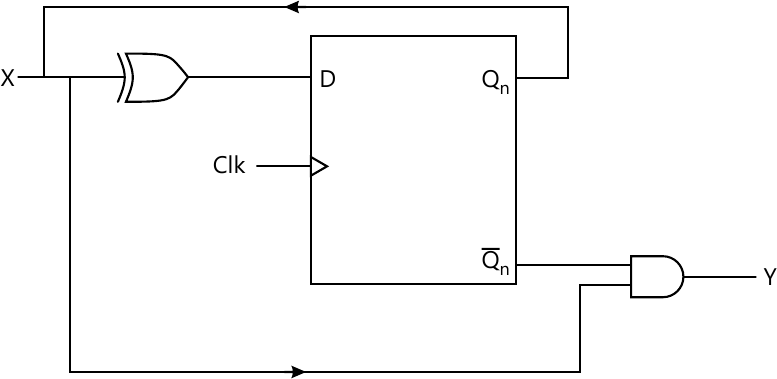MORE IN Analog and Digital Electronics
VTU Computer Science (Semester 3)
Analog and Digital Electronics
December 2012
Total marks: --
Total time: --
INSTRUCTIONS
(1) Assume appropriate data and state your reasons
(2) Marks are given to the right of every question
(3) Draw neat diagrams wherever necessary

1 (a) Differentiate analog and digital signals. Define period, frequency and duty cycle of a digital signal. Prove that the duty cycle of a symmetrical waveform is 50%.
6 M
1 (b) What are the universal gates? Realize ((A+B)⋅ C) using only NAND gates.
5 M
1 (c) Describe positive and negative logic. List the equivalences between them.
4 M
1 (d) What is the need for HDL? Explain the structure of VHDL/verilog program.
5 M

2 (a) Find the minimal SOP of the following Boolean functions using K-Maps:
i) f(a,b,c,d)=∑m(6,7,9,10,13)+d(1,4,5,11)
ii) f(w,x,y,z)=πm(1,2,3,4,9,10)+d(0,1,4,15)
8 M
2 (b) Simplify f(A, B, C, D)=∑m(0,1,2,3,10,11,12,13,14,15) using Quine-Mc Cusky method.
8 M
2 (c) What are static hazards? How to design a hazard free circuit? Explain with an example.
4 M

3 (a) Design and implement BCD to excess-3 code converter using four 8:1 multiplexers. Take MSB 'A' as map enterd variable (input variable) 'BCD' lines as select lines, assuming f(A,B,C,D) as BCD input.
8 M
3 (b) Realize a logic circuit for Ocatl to binary encoder.
6 M
3 (c) Draw the PLA circuit and realize the Boolean functions: X=A'B'C+AB'C'+B'C, Y=A'B'C+AB'C', Z=B'C
4 M
3 (d) Give the HDL implementation of 2:1 MUX.
2 M

4 (a) Draw the logic diagram, truth table and timing diagram for edge-triggered D-flip flop.
6 M
4 (b) With a neat logic diagram and truth table, explain the working of JK Master-Slave Flip-Flop along with its implementation using NAND gates.
6 M
4 (c) Analyze the behavior of the sequential circuit shown in Fig.Q4(c) and draw the state table and state transition diagram.8 M

5 (a) Using positive edge triggered D flip flops, draw the logic diagram for a 4-bit parallel-in-serial-out (PISO) shift register and explain its workign to load 1001 into it and shift the same.
8 M
5 (b) With a neat diagram, explain how shift registers can be applied for serial that operates at clock frequency of 5 MHz. Also, what is the time required to extract 4-bit number from PISO operates at 5 MHz clock?
4 M
5 (c) Write verilog/VHDL, code for Johnson counter.
3 M

6 (a) With the help of neat block diagram and timing diagram, explain the working of a Mod-16 ripple counter constructed using positive edge triggered JK flip-flops.
8 M
6 (b) Design a self-correcting Mod-5 synchronous down counter using JK flip-flops. Assume 100 as the next state for all the unused states.
8 M
6 (c) List any two drawbacks of asynchronous counter. What is the clock frequency in a 3-bit counter, if the clock period of the waveform at last flip-flop is 24 μs?
4 M

7 (a) How does state transition diagram of Moore machine differ from Mealy machine? Draw the state transition diagram of synchronous sequential logic circuit using Mealy model that defects three consecutive zeros from an input data stream, X and signals detection by making output Y=1.
6 M
7 (b) Draw the ASM chart vending machine problem using Mealy model.
8 M
7 (c) What is the use of state reduction technique? Demonstrate the state reduction by implication table method.
6 M

8 (a) With a neat diagram, explain the working of a 4-bit D/A converter.
8 M
8 (b) What is the accuracy and resolution of an 8-bit D/A converter? Find the resolution and accuracy of the same if the full scale output is +10V.
4 M
8 (c) Discuss the working of following A/D converters:
i) Successive approximation A/D.
ii) Counter type A/D.
8 M

More question papers from Analog and Digital Electronics